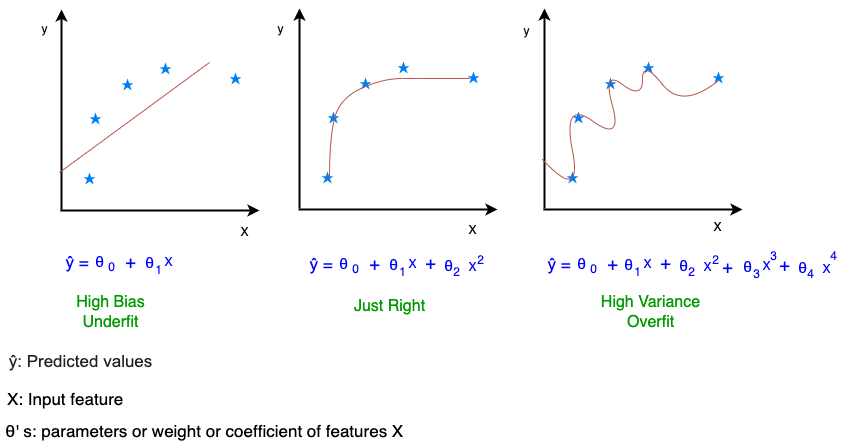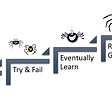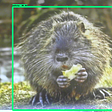# Regularization in Machine Learning

1. Overfitting:

Sometimes the machine learning model performs well with the training data but does not perform well with the test data. It means the model is not able to predict the output when dealing with unseen data by introducing noise in the output, and hence the model is called overfitting. Noise means the data points that do not represent the actual property of the data, but random chance.

2. Overfitting Examples:

In Image1, we are trying to fit a model on regression data. We can use one of the linear, quadratic, and polynomial function while fitting a regression model. Many times a linear regression model may under fits the data while a quadratic function form may provide a better fit. To derive a greater performance of fit, we can use a polynomial function which will fit the data very closely. While polynomial function fitting may appear a great model for this dataset, but if we change the dataset, the same model may turn out to be a poor fit for the new data (i.e. high variance). This is because the polynomial function fits the model so close to the original data that it does not generalizable across other similar data, which would result in overfitting.Image1: Under fit — Linear function(Left), Just Right — Quadratic function (Center), Overfit — Polynomial function (Right) fitting to the regression data

In Image2, when we try to fit a model on classification data. Linear function would be too simple to explain variance in the data and hence result in Under fit. Quadratic function form may result in an appropriate fit. The polynomial function form would predict too good to be true, and it might fail to perform well on unseen data and result in overfitting.

3. How to overcome Overfitting?

One way is to reduce the number of features in the model. But doing so would result in loss of information, and thus the model will not have the benefit of all the information (provided by features) that is available.

When we have a lot of features and each feature contributes a bit to predict, we can’t remove the features. In this case, the solution is Regularization. Our model needs to be robust to perform well on both train and test data. For that, we try to:

• Shrink the coefficient (or weight or parameter θ) of the features in the model
• Getting rid of high degree polynomial features from the model

The above solution would result in a simpler hypothesis and be less prone to overfitting. This can be achieved using Regularization. This technique discourages the learning of a more complex or flexible model, to avoid the risk of overfitting.

4. What parameters (θ’s) to penalize?

Now we know that in the Regularization technique we reduce the magnitude/value of features (called θ’s) and penalize/ reduce the impact of higher-degree polynomial terms of features. But we don’t know which parameters (θ’s) are high order degree polynomial terms. So in Regularization, we are going to modify the cost function to shrink all the parameters (θ’s).

5. Regularization:

It is a technique to prevent the model from overfitting by adding extra information to it. During Regularization, the predicted output function does not change. The change is only in the cost function.

The cost function of Linear Regression which is called Residual Sum of Square (RSS) is given by:

Based on the training data, RSS will adjust the coefficient θs to minimize the cost function using Gradient Descent (or other optimization techniques). If there is noise in the training data, the model will not be able to generalize well to the future unseen data and will overfit. Here, Regularization comes into the picture and shrinks these coefficients to zero.

6. Types of Regularization:

Regularization could be of types:

L1 Norm or Lasso Regression

L2 Norm or Ridge Regression

• L1-Lasso Regression helps to reduce the overfitting in the model as well as feature selection. The L1 penalty forces some coefficient estimates to be exactly equal to zero, which means there is complete removal of some features for model evaluation when the tuning parameter lambda (λ) is sufficiently large. Therefore, the lasso method also performs feature selection and is said to yield sparse models.
• L2-Ridge Regression is mostly used to reduce the overfitting in the model, and it includes all the features present in the model. It reduces the complexity of the model by shrinking the coefficients. The cost function is altered by adding the penalty term (shrinkage term), which multiplies the lambda (λ) with the squared weight (θi) of each individual feature. The penalty term regularizes the coefficients of the model, and hence ridge regression reduces the magnitudes of the coefficients that help to decrease the complexity of the model. The cost function becomes:

λ is the regularization parameter, which decides how much to penalize the flexibility of the model. If the model is highly flexible which means the variance of the model is very high, and it changes with a small amount of change in the data → coefficient of the model would be larger. But in order to minimize the cost function (with regularization), these coefficient values should be less. That’s how Ridge Regularization prevents the coefficient values from rising too high.

Here we are not penalizing θ0 and penalty is starting from θ1, in practice it makes very little difference in the final result, so by convention, we only penalize coefficient starting from θ1 till θp.

When λ = 0, Ridge Regularization will not do any regularization, the model will remain overfitting and with high variance.

When λ is very large → infinity → loss term is diminished → the training data does not participate in the optimization → we are just optimizing for the regularization term, the cost function is minimized when θ1, θ2, …, θp all = 0 → cost function remains only with bias term-b → results in linear model → Under fitHigh Bias

Selecting the good value of λ is critical, the selection is done using cross-validation.

7. Regularization Parameter λ:

λ is a regularization parameter that controls overfitting, and It's the tradeoff between 2 terms:

• Fitting training dataset well
• Keeping parameters θ’s small and keeping hypothesis simple to avoid overfitting

8. Why L1 creates Sparsity?

With a sparse model, we think of a model where many of the weights /coefficients (θ’s) are 0. Let us therefore reason about how L1 is more likely to create 0 weights. If we compare the cost function (with regularization term) for L2:

and L1:

In optimization formulation for comparison, Loss and λ can be ignored as they are the same for both L1 and L2 regularization. So we end up comparing below:

which are of shown diagrammatically as follows:

Derivative of L2 and L2 is represented as below:

Weight updates for both L2 and L1 is given by below formula of Gradient Descent:

Let θ1 is positive (similarly can we can do for negative θ1):

L2 updates occurs less when compared to L1 updates as we reach closer to optimum. That is, the rate of convergence decreases because in L2 regularization we have 2 * θ1 *α, which is less than α. L2 doesn’t change the value of θ1 from one iteration to another. L1 regularization continues to constantly reduce θ1 towards θ1 = 0. This happens because L1 derivative is constant and L2 derivative is not constant. The chance of weights reaching 0 is more for L1 regularization, as the derivative is constant and independent of the previous weight value. L2 regularization has derivatives reducing as the derivative is dependent on the previous iteration weight value, which is converging to optimal.

9. References:

--

--

--

## More from Heena Sharma

Love podcasts or audiobooks? Learn on the go with our new app.

## Optimizing network traffic in a massively parallel real-time bidding environment## A Gentle Introduction to Reinforcement Learning## Build your Machine Learning models the easy way with SPSS## Object detection with TensorFlow on Raspberry Pi## NLP Newsletter: Reformer, DeepMath, ELECTRA, TinyBERT for Search, VizSeq, Open-Sourcing ML,…## Common PyTorch Functions## How to Add Regularization to Keras Pre-trained Models the Right Way## Machine Learning Experiments for Making Model the Right Fit## Naive Bayes in it’s easiest form## Random forests for variable importance 🌲🌳🎄## Activation functions and its types## Decision Trees and Random Forests.